Li, J., Lu, Y., Peng, X., Jiang, P., Zhang, B., Zhang, L., Meng, H., Kan, Z., and Wang, X. (2022). "Discrete element method for simulation and calibration of cotton stalk contact parameters," BioResources 18(1), 400-416.

#### Abstract

To improve the accuracy of the discrete element research, physical and simulation experiments were used to calibrate the cotton stalk contact parameters. Based on the stalk-stalk and stalk-steel contact mechanics, the parameters were measured in physical experiments, and the discrete element simulation software was used to build the stalk model. In the simulation process, the Plackett-Burman experiment was used to screen three significant factors from six initial factors. The steepest Plackett-Burman experiment was used to determine the optimal interval of the significant factors. A second-order regression model of the significant factors and the angle of repose was established according to the Central Composite design experiment. The best parameter combination of the significant factors was then obtained: the coefficient of static friction on stalk-steel contact was 0.31, the coefficient of static friction on stalk-stalk contact was 0.62, and the coefficient of rolling friction on stalk-stalk contact was 0.02. The relative error between the physical angle of repose and the simulated angle was 3.27%, indicating that it is feasible to apply the simulation experiment instead of the physical one. It offers insights into cotton stalk contact parameter settings and film-stalk separation in the simulation.

Discrete Element Method for Simulation and Calibration of Cotton Stalk Contact Parameters

Jiali Li,a Yongtao Lu,c Xiangbin Peng,a Peng Jiang,a Bingcheng Zhang,a Lanyu Zhang,a Hewei Meng,a,* Za Kan,a,* and Xinzhong Wang b

To improve the accuracy of the discrete element research, physical and simulation experiments were used to calibrate the cotton stalk contact parameters. Based on the stalk-stalk and stalk-steel contact mechanics, the parameters were measured in physical experiments, and the discrete element simulation software was used to build the stalk model. In the simulation process, the Plackett-Burman experiment was used to screen three significant factors from six initial factors. The steepest Plackett-Burman experiment was used to determine the optimal interval of the significant factors. A second-order regression model of the significant factors and the angle of repose was established according to the Central Composite design experiment. The best parameter combination of the significant factors was then obtained: the coefficient of static friction on stalk-steel contact was 0.31, the coefficient of static friction on stalk-stalk contact was 0.62, and the coefficient of rolling friction on stalk-stalk contact was 0.02. The relative error between the physical angle of repose and the simulated angle was 3.27%, indicating that it is feasible to apply the simulation experiment instead of the physical one. It offers insights into cotton stalk contact parameter settings and film-stalk separation in the simulation.

DOI: 10.15376/biores.18.1.400-416

Keywords: Cotton stalk; Discrete element simulation; Parameter calibration; Angle of repose

Contact information: a: College of Mechanical and Electrical Engineering, Shihezi University, Shihezi, 832000, P.R. China; b: School of Agricultural Equipment Engineering, Jiangsu University, Zhenjiang, 212013, P.R. China; c: Institute of Machinery and Equipment, Xinjiang Academy of Agricultural Reclamation, Shihezi, 832000, P.R. China; *Corresponding author: kz-shz@163.com; mhw_mac@shzu.edu.cn

INTRODUCTION

Polyethylene mulching is an important measure of cotton yield enhancement. The mulch film is laid before cotton sowing and recovered after harvest every year. Agricultural mulch film has poor mechanical properties and breaks severely after the tillage period, forming a residual film. Residual film is a plastic waste, which is not easy to decompose. The accumulation of residual film in soil will change the physical and chemical properties of the soil, which is a threat to the surrounding ecology (Hu et al. 2019). If the recovered film is not promptly dealt with, it will also pollute the air and soil environment (Hu et al. 2019). Currently, the main problem that restricts the resource utilization of residual film is the high content of impurities. Cotton stalk is the main component in the mixture of residual film and impurities. Thus, it is particularly important to explore the movement law of cotton stalk in the separation process for the design of separation device.

In recent years, discrete element simulation software has become more and more widely used in the field of agricultural materials (Boac et al. 2010; Petingco et al. 2020). Numerically simulating the film stalk separation process by using this method is helpful to reveal the film stalk screening mechanism (Gao et al. 2017; Chen et al. 2019). To improve the accuracy of the discrete element model of cotton stalk, it is necessary to define the intrinsic parameters and contact parameters precisely (Zhang et al. 2019; Nguyen et al. 2021). The contact parameters mainly include the coefficient of static friction, the coefficient of rolling friction, and the coefficient of restitution (Coetzee 2020; Ma et al. 2020).

Many researchers have used the discrete element method to calibrate the material contact parameters, mainly in the research of crop stalks and seeds. In terms of crop stalks, Zhang et al. (2018) used the bucket-discharged method to measure the angle of repose of the corn stalks and used the orthogonal experiment to calibrate the contact parameters. Liao et al. (2020) used the cylinder lifting method to measure the angle of repose of the forage rape stalks. The contact parameters were calibrated by combining the two-level factor experiment and the response surface experiment. Wang et al. (2022) used the Plackett-Burman and the Box-Behnken Design experiments to calibrate the parameters of alfalfa based on the angle of repose. Zhang et al. (2020) calibrated the key parameters of the corn stalks crushing process based on the Hertz-Mindlin with bonding contact model. In terms of seeds, the contact parameters of castor bean capsules were calibrated by establishing a three-dimensional model and using the discrete element method to design a Central Composite Design experiment (Hou et al. 2019). Virtual design of experiment (DOE) was used to change corn contact parameters for simulation calibration (Mohammad et al. 2017).

The above-mentioned studies showed that the application of the discrete element methods to agricultural materials has been explored extensively. However, few studies have been done on cotton stalk. The cotton stalk is severely lignified and shows significant differences in material properties from the stalk of crops such as corn, forage rape, and sugarcane. Therefore, the calibration of contact parameters is important when using the discrete element method for the cotton stalk motion process study.

In this study, the cotton stalk of Xinluzao No.83 variety was selected as the research object. The relevant contact parameters of the stalk were measured through physical experiments. The angle of repose of the stalk was obtained by the bucket-discharged experiment, and the relative error of the angle was used as the response index. The discrete element method was used to study the mechanical contact characteristics of stalk-stalk and stalk-steel. The contact parameters in the Experts in Discrete Element Modeling (EDEM) software were calibrated through the Plackett-Burman, the steepest Plackett-Burman, and the Central Composite Design experiments. The calibrated cotton stalk contact parameters can be used for the discrete element method to simulate the movement law of cotton stalks on the separation device.

MATERIALS AND METHODS

Physical Experiments

Xinluzao No. 83, one of the cotton varieties grown in Xinjiang, was used as the research material. The experimental cotton stalks were sampled from a cotton field at Shihezi University, Xinjiang and stored in the natural state after manual harvesting in autumn. The sampling site was near the middle and lower parts of the cotton plant, about 10 cm above the stubble. About 500 stalks were cut with pruning shears (Xiong Yue San Huan Horticulture tools Co., Ltd, Liaoning, China). They were characterized by a uniform length of 80 mm for straight and disease-free branches. The digital Sartorius MA100d electronic moisture analyzer (Sartorius, Göttingen, Germany) was used to measure the moisture content. The moisture content accuracy of the analyzer was 0.01%, and the quality accuracy was 0.001 g. The average moisture content of the stalks was 17.74%. The digital vernier caliper with an accuracy of 0.02 mm was used to measure the stalk diameters. The average diameters of the upper, middle, and lower ends of the stalks were 9.05 mm, 9.33 mm, and 9.38 mm, respectively.

Cotton stalk physical properties

The coefficient of static friction μs of the cotton stalk was determined by the inclined method (Gu et al. 2017), and the instrument was a friction angle tester (Shihezi Univesity, China), as shown in Fig. 1. Two stalks were fixed as a group as the experimental object (Zhang et al. 2017). To avoid gaps between the experimental stalks and the contact surface, the difference in the average diameter of the same group was required to be less than 5%. The coefficient of static friction on stalk-steel contact μs(k-l) was measured when the contact surface was steel. The coefficient of static friction on stalk-stalk contact μs(k-k) was measured when the contact surface was stalk bark. The bark was peeled off from the cotton stalk and pasted on the surface of the inclined plane of the friction angle tester. During the experiment, a set of samples were placed on the upper part of the inclined plane. The inclination angle of the plate was gradually increased by turning the handwheel. When the stalk sample was slightly moved, the angle of the plane at this moment was recorded as the sliding friction angle. According to Eq. 1, μs(k-l) and μs(k-k) were calculated (Wen et al. 2017). The experiment was repeated 50 times, and the average of the calculation results was taken. Here is the expression of Eq. 1,

tan(α) = f                       (1)

where α is the sliding friction angle (°), and f is the coefficient of static friction.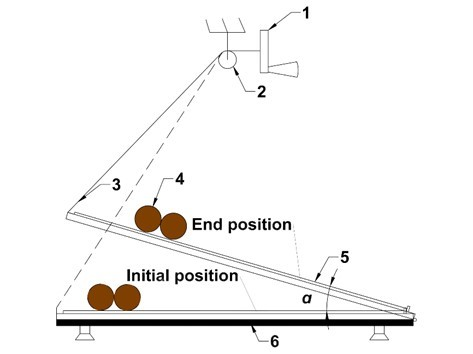Fig. 1. The tester of angle of friction: 1: Handwheel; 2: Lifting device; 3: Tow rope; 4: Cotton stalk samples; 5: Replaceable board; 6: Base

The device for measuring the coefficient of rolling friction μk of cotton stalk was the same as the coefficient of static friction μs. The difference between the measurement methods was that the former was measured with a single cotton stalk (Wen et al. 2020a). To reduce the influence of the burrs at both ends of the cut stalk on the rolling, the burrs were lightly polished off with sandpaper. The friction angle tester was adjusted to the horizontal state, the stalk sample was placed on the tester, and the inclination angle of the tester was changed. The angle was recorded as the rolling friction angle when the stalk sample started to roll. The experiment was repeated 50 times, and the average value was taken.

The coefficient of restitution on stalk-steel contact μr(k-l) and that on stalk-stalk contact μr(k-k) were measured, as shown in Fig. 2. The μr(k-l) and μr(k-k) were calculated by simplified Eq. 2 (Liu et al. 2020; Wang et al. 2020). Here is the expression of Eq. 2,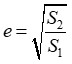(2)

where e is the coefficient of restitution, S1 is the initial drop height of sample (mm), and S2 is the rebound height of sample (mm).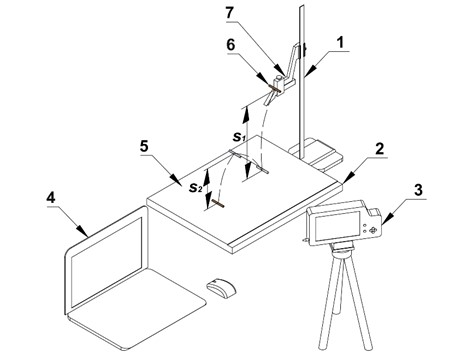Fig. 2. The measurement device of the coefficient of restitution: 1: Movable height gauge; 2: Collision base; 3: High-speed camera; 4: PC-Computer; 5: Replaceable collision contact surface; 6: Cotton stalk sample; 7: Loading platform. Note: S1 is the initial drop height of sample (mm), and S2 is the rebound height of sample (mm)

When the stalks fall, the windward area is small and the speed is fast, and the air resistance can be ignored. Five release heights of 300, 350, 400, 450, and 500 mm were selected. Each stalk sample was placed on the measuring device and parallel to the outer edge of the release table. According to the requirements of measuring μr(k-l) and μr(k-k), the falling plane was evenly laid with corresponding materials to ensure that the cotton stalk contact surface was flat. When the sample was in free fall, the high-speed camera (Fastec Imaging, FASTEC TS4, USA) was used to shoot its trajectory. The initial drop height and rebound height of the sample were recorded, named, and stored. High-speed camera analysis software ProAnalys (Fastec Imaging, FASTEC TS4, USA) was used to process the experiment data. The height ruler was taken as the reference system. When the cotton stalk was at the initial height, its projection center on the contact surface was taken as the origin. The X-Y coordinate system was established to obtain the movement trajectory of the sample’s falling rebound over time. The coordinate values in the high-speed camera analysis software were imported into the Origin 2019b software (OriginLab, Northampton, MA, USA). The time-displacement image of the cotton stalk falling process was obtained. The experiment was repeated 5 times at each height to find the average value.

Angle of repose experiment

The angle of repose was measured by the bucket-discharged method, which was similar to the method used in the study by Mohammad et al. (2017). The length of the stalks was 80 mm. Based on this, a device with a steel extractable gate was designed, as shown in Fig. 3. The device was made of plexiglass, with a steel plane 400 mm long and 100 mm wide at the bottom, surrounded by plexiglass panels with a height of 200 mm, and equipped with gate slots. The gate was inserted, and the device was filled with the stalks. When the gate was removed, the stalks moved. After the stalks stopped moving, the angle between the inclined surface of the stalk material flow and the bottom surface was seen as the angle of repose. The experimental results were photographed by the camera and processed by Matlab R2015b software (Mathworks, Massachusetts, USA). The digital image tool of Origin 2019b was used to delimit the pixel value range of the image, extract the boundary curve, perform linear fitting, calculate the slope of the fitted curve, and obtain the angle values (Wen et al. 2020b; Yu et al. 2020). The experiment was carried out 20 times, and the average value was taken.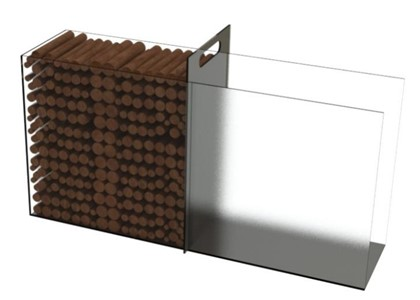Fig. 3. The measuring instrument of angle of repose

Simulation Experiments

A method that combined the physical and simulation experiments was used to calibrate the cotton stalk contact parameters. The angle of repose θ of the cotton stalks was measured by the bucket-discharged method (Tekeste et al. 2018). The EDEM 2021 software (Altair, Troy, Mich., USA) was used to simulate the angle. The Design-Expert 8.0.6 software (Stat Ease Inc., Minneapolis, USA) was used to design the Plackett-Burman experiment, the steepest Plackett-Burman experiment, and the Central Composite Design experiment. Through the Plackett-Burman experiment, the significant factors were determined. Through the steepest Plackett-Burman experiment, the level range of the significant factors was narrowed. The optimal parameter combination of the significant factor was obtained through the Central Composite Design experiment. The angle under the optimal combination was compared with the physical. Then, the simulation experiment was optimized.

Modeling and parameter setting

Firstly, the standard single sphere particles were selected as the basic unit of the discrete element model of the cotton stalk. The particle radius was 1.0 mm. The stalk model was formed by stacking 40 particle layers, with an angle difference of 30° between the particle positions of two adjacent layers. Each particle layer contained 18 single-sphere particles (Fig. 4). The x-axis, y-axis, and z-axis coordinate positions of these 720 particles were calculated and input into EDEM 2021 software to generate a cylindrical model with a diameter of 10 mm and a length of 80 mm. The basic parameters of the model were set according to the intrinsic parameters of the cotton stalk, as shown in Table 1 (Jiang et al. 2019). Secondly, the angle of repose measurement device model was imported into EDEM 2021 software, and its material property was steel. The basic parameters of the device model were set according to the steel. The particle factory was established in the device model, and the number of stalk model was set to 454.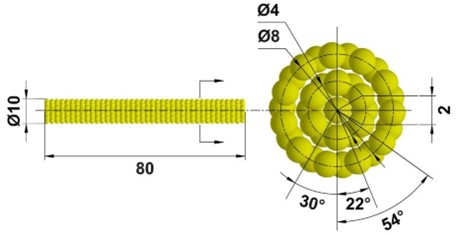Fig. 4. Discrete element model of cotton stalk. Note: The unit for all numbers was mm.

Table 1. Material Parameter Settings of Discrete Element Method Simulation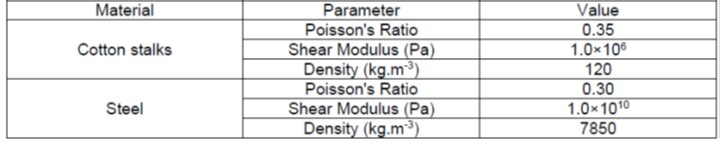Then, the contact parameters were set according to the experiment scheme. The Hertz-Mindlin contact model is one of the most commonly used models to simulate the behavior of non-viscous particles. The Hertz-Mindlin (no-slip) contact model is a nonlinear spring elastic model without cohesion. Therefore, it was selected for the stalk-stalk and stalk-steel contact model. The body-centered cubic form (bcc) was selected for the accumulation of stalks. The Rayleigh time step was set to 35 %, the simulation time was 50 s, the extraction speed was 50 mm/s (Hou et al. 2019), and the extraction start time was 15 s. Finally, the simulation started. After the extraction of the gate, the stalks changed from a static stable state to a natural accumulation state and formed an angle.

In EDEM 2021 software, the color was used to represent the movement change of the stalk models, and the blue indicated that the models were in a static state, as shown in Fig. 5. The figure was saved, and the accumulation angle was extracted through the Matlab R2015b software to obtain the angle of repose.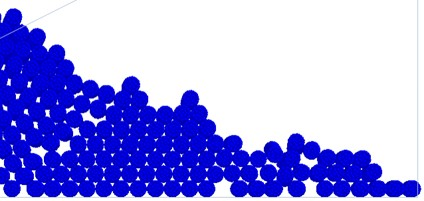Fig. 5. The natural accumulation state of the cotton stalk model

Plackett-Burman experiment

The Plackett-Burman experiment is a screening design experiment. It is suitable for experimental design under the condition of many experimental factors, aiming to screen out the factors that have significant influence. The six contact parameters to be screened were: the coefficient of static friction on stalk-steel contact μs(k-l), the coefficient of static friction on stalk-stalk contact μs(k-k), the coefficient of rolling friction on stalk-steel contact μk(k-l), the coefficient of rolling friction on stalk-stalk contact μk(k-k), the coefficient of restitution on stalk-steel contact μr(k-l), and the coefficient of restitution on stalk-stalk contact μr(k-k). Three parameters, which had significant effects on the response value, were selected.

The experimental factors and levels were set as shown in Table 2. In the Plackett-Burman experiment, the selection of the levels of the factors is not clearly defined, and the appropriate level can be set according to the actual situation. The length of the cotton stalk model was 80 mm, and the contact area with the device material was large. The model was composed of multiple spherical particles, which had increased surface roughness compared to the actual one. Therefore, the range of parameter levels was set based on 0.5 to 1.1 times the measured average value. In total, two levels and three center points were set, and 15 experiments were performed.

Table 2. Plackett-Burman Experimental Factors and Levels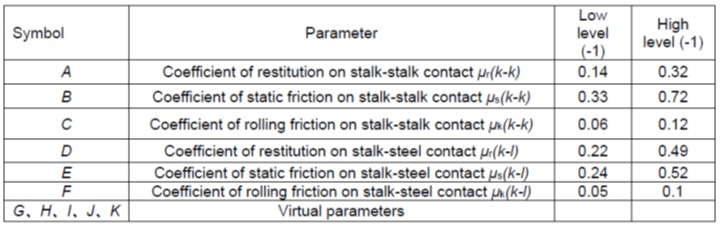Steepest Plackett-Burman experiment

To make the level of each factor approach the area near the optimal value as soon as possible, the steepest Plackett-Burman experiment was carried out based on the Plackett-Burman experiment. In this design, three factors were set. The level of significant factors was centered on the measured average value, and five levels were set in equal steps. The coefficient of rolling friction on stalk-stalk contact μk(k-k) was larger than the reference value (Jiang et al. 2019), so the lowest level of the Plackett-Burman simulation experiment was set as the center, and five levels were set with equal steps. The non-significant factor level was set according to the average value of the physical measured contact parameters, and the simulation experiment was performed with the angle of repose of physical experiment θs as the experimental index. A total of five experiments were performed.

Central Composite Design experiment

The Central Composite Design experiment was used to obtain the optimal parameter combination. The optimal area of each significant factor was determined through the steepest Plackett-Burman experiment, and five levels of significant experimental factors with this area were set as the center. The average value of the physical experiment was taken as the non-significant factors, and six center points were used to carry out the Central Composite Design experiment. A total of 20 experiments were performed. The response surface analysis was carried out, the regression equations of factors and response values were obtained, and optimal combination prediction of significant factors was made.

RESULTS AND DISCUSSION

Cotton Stalk Physical Properties

The sliding friction angles of stalk-steel and stalk-stalk were 25.32° and 32.89°, respectively. Correspondingly, the coefficient of static friction on stalk-steel contact μs(k-l) and that on stalk-stalk contact μs(k-k) were 0.47 and 0.65.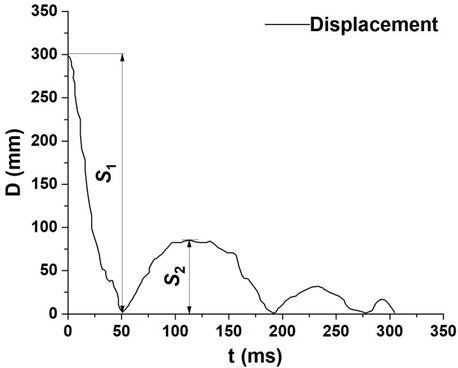Fig. 6. Time-displacement curve of cotton stalks movement

Angle of Repose Experiment

The image of the angle of repose was obtained. The Matlab R2015b software was used to process the original image with gray scale and binarization. The holes were filled, and the stalk boundary curve was extracted, as shown in Fig. 7.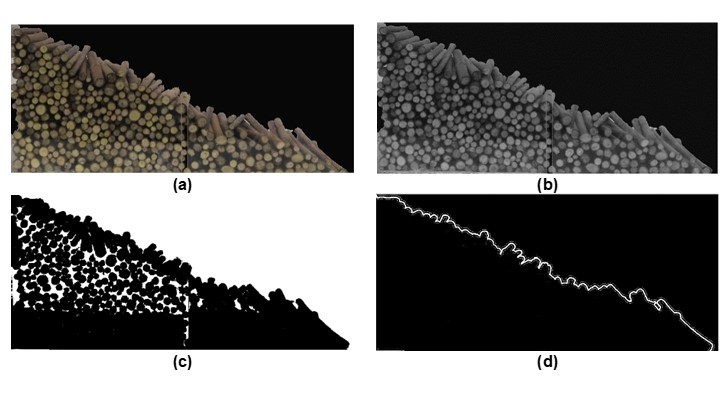Fig. 7. The angle of repose of the cotton stalk image processing steps: (a): original image; (b): gray processing; (c) binarization processing; and (d): hole filling

Figure 8 is an example of fitting curve, and the angle between the fitting curve and the horizontal axis is the angle of repose. The average value of θp obtained was 24.79°.

The governing factors for the angle of repose were analyzed. In the initial state, the cotton stalks were in a stable and static state under the action of the side wall and the gate. The stalks in contact with the gate were directly subjected to the side pressure of the gate. The stalks without contact with the gate were indirectly subjected to the lateral pressure. The effect of the pressure existed in the form of lateral extrusion of the stalks to stalks, and gradually weakened in the direction far from the gate (L was close to zero). When the gate was evacuated, the side pressure disappeared, and the stalks moved under the action of downward gravity, lateral inertia, and friction between materials until the force was balanced. At this time, the stalks stopped moving and formed the angle.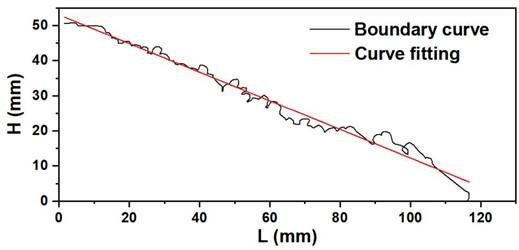Fig. 8. Linear fitting by using Origin 2019b software

Plackett-Burman Experiment

The codes and results of the Plackett-Burman experiment factors are shown in Table 3, and the analysis of variance (ANOVA) of the experimental factors are shown in Table 4. From the ANOVA results in Table 4, the P-values for the coefficient of rolling friction on stalk-stalk contact μk(k-k) (C), the coefficient of static friction on stalk-steel contact μs(k-l) (E), and the coefficient of static friction on stalk-stalk contact μs(k-k) (B) were less than 0.05, which were all significant factors.

Table 3. Design and Results of Plackett-Burman Experiment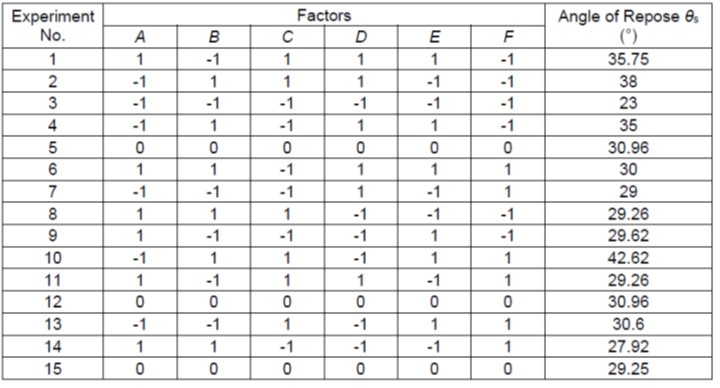Table 4. ANOVA of Plackett-Burman Experiment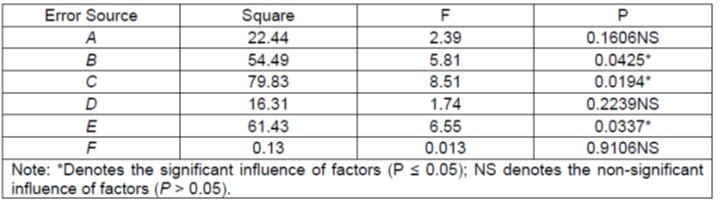Steepest Plackett-Burman Experiment

As shown in Table 5, the coefficient of rolling friction on stalk-stalk contact μk(k-k), the coefficient of static friction on stalk-steel contact μs(k-l), and the coefficient of static friction on stalk-stalk contact μs(k-k) decreased with their respective levels. The relative error between the simulated value and the physical value of the angle of repose θ was also reduced. The error was reduced to the lowest in the No. 4 experiment, and the result was 12.5%. Therefore, the center points of μk(k-k), μs(k-l), and μs(k-k) were set to 0.04, 0.37, and 0.55, respectively, and the Central Composite Design experiment was performed.

Table 5. Design and Results of Steepest Plackett-Burman Experiment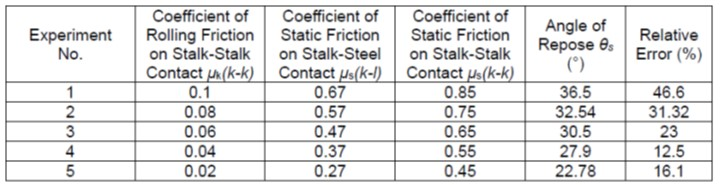Central Composite Design Experiment

Experiment results

The level of each experimental factor is shown in Table 6, and other levels were set according to the default values of Design-expert 8.0.6 software. A three-factor five-level Central Composite Design experiment was carried out, and six central points were selected for a total of 20 groups of experiments. Table 6 is the Central Composite Design experimental factor level setting, and Table 7 is the Central Composite Design experiment design and results.

Table 6. Central Composite Design Experiment Factors and Levels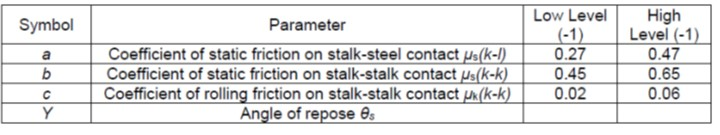Table 7. Design and Results of Central Composite Design Experiment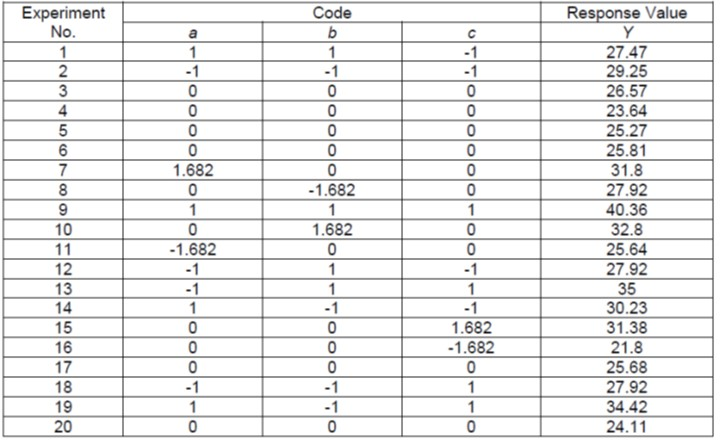Regression model establishment and significance analysis

Table 8 shows that the F-value of the model was 12.28. The larger the F-value, the more obvious is the effect between treatments. The coefficient of variation (CV) was 6.22%, which indicated that the experiment had higher reliability. The AdepPrecisior-value was 12.715, which proved that the model had higher accuracy. The coefficient of determination R2 of the equation was 0.9170, which was close to the upper limit of the value range of 0 to 1. The second-order regression model fitted well. The adjusted R12-value was 0.8424, which was close to the value of R2, indicating that the model was highly significant. It is considered that the model curve simulation experiment value was more authentic, and the quadratic polynomial equation of the significant factor on the angle of repose θs was obtained as Eq. 3,

Y = 25.09+1.67a+1.25b+2.85c-0.32ab+1.42ac+2.14bc+1.84a2+2.41b2+1.09c2 (3)

Table 8. ANOVA of Central Composite Design Experiment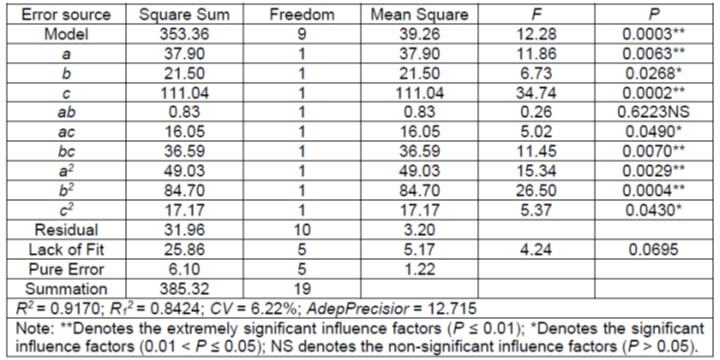The P-values of the single factor items a and c and the interaction items bc, a2, and b2 were less than 0.01, which means that they had an extremely significant influence on the response value Y. The P-values of the single factor item b and the interaction items ac and c2 were greater than 0.01 and less than 0.05, which had a significant influence on the response value Y. In the ANOVA, the smaller the P-value, the more significant the factor was. From the P-values of the above factors, the order of influence of each factor was: c > b2 > a2 > a > bc > b > c2 > ac. The P-value of interaction item ab was much larger than 0.05, which was an unobvious factor to the response value Y. Therefore, if the non-obvious influencing factors in the second-order response model were eliminated, Eq. 3 can be changed to Eq. 4,

Y = 25.09+1.67a+1.25b+2.85c+1.42ac+2.14bc+1.84a2+2.41b2+1.09c2 (4)

Interaction analysis

Based on the above variance analysis, the interaction items ac and bc that had a significant influence on the response value Y were analyzed. When μs(k-k) (b) was set to the central level (0.55), the interaction between μs(k-l) (a) and μk(k-k) (c) was analyzed. It can be seen from Fig. 9 that as μs(k-l) (a) increased from 0.27 to 0.47, θs decreased first and then increased. When μs(k-l) (a) was greater than 0.32, θs no longer decreased. As μk(k-k) (c) increased in the range of 0.02 to 0.06, θs also gradually increased. The response surface curve changed faster along the μk(k-k) (c) direction. At the central level, the effect of μk(k-k) (c) on θs was more significant than that of μs(k-l) (a).

When μs(k-l) (a) was set to the central level (0.37), the interaction between μs(k-k) (b) and μk(k-k) (c) was analyzed. It can be seen from Fig. 10 that as μs(k-k) (b) increased in the range of 0.45 to 0.65, θs decreased first, and then increased. When μs(k-k) (b) was greater than 0.55, θs no longer decreased. As μk(k-k) (c) increased from 0.02 to 0.06, θs also gradually increased. The response surface curve changed faster along the μk(k-k) (c) direction. At the central level, the effect of μk(k-k) (c) on θs was more significant than that of μs(k-k) (b).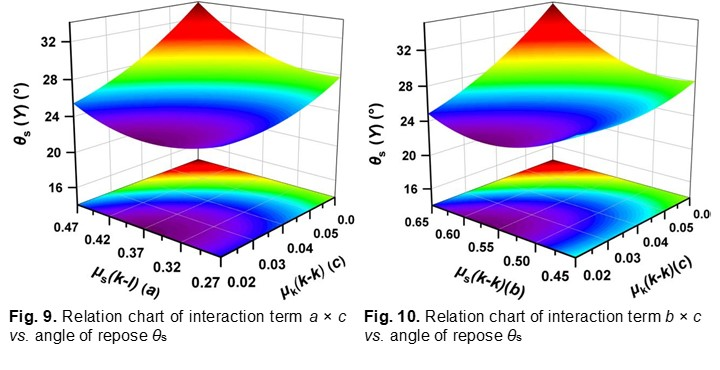Parameter Optimization and Experiment Verification

Parameter optimization

According to the experiment results, the optimization in Design-expert 8.0.6 software was used to optimize the parameter combination. The constraint conditions of each experiment factor were: the coefficient of static friction on stalk-steel contact μs(k-l) ranged from 0.27 to 0.47, the coefficient of static friction on stalk-stalk contact μs(k-k) ranged from 0.45 to 0.65, and the coefficient of rolling friction on stalk-stalk contact μk(k-k) ranged from 0.02 to 0.06.

The target value of response index Y was set to the angle of repose of physical experiment θp (24.79°). The optimization results of the three factors were: μs(k-l) was 0.31, μs(k-k) was 0.62, and μk(k-k) was 0.02. The response value of the surface of the model was better, and the predicted angle of repose was 24.41°.

Experiment verification

To verify the accuracy of the model prediction and the reliability of the parameter optimization combination, the above parameter combination was used for 3 experiments in the discrete element simulation software. The other three parameters were set according to the physical measured values. The optimal combination of experiment parameters was shown in Table 9, and the results were shown in Table 10.

Table 10 shows that the simulation results were close to the physical experiment results. The relative error between the angle of repose of simulation experiment θs and the angle of repose of physical experiment θp was less than 5%. Thus, the parameter optimization model and parameter combination were reliable, which can provide theoretical support for the discrete element simulation of cotton stalk.

Table 9. Optimal Combination of Experiment Parameters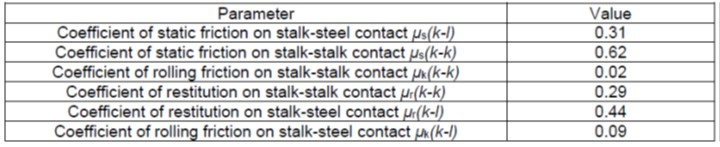Table 10. Comparison between Model Optimization and Physical Experiment